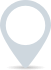# Notional Value

Notional value, in financial terms, refers to the theoretical or nominal value of a financial instrument or contract. It represents the value of an asset or liability based on the face value or the stated amount, without considering factors like market conditions or risk.

Notional value is commonly used in various financial contexts, including:

1. Derivatives: In derivatives markets, such as futures and options, the notional value is the value of the underlying asset that the contract is based on. For example, in a futures contract for 100 barrels of oil, the notional value would be the current market price of 100 barrels of oil.

2. Swaps: In financial swaps, the notional value is the specified principal amount on which the interest rate or cash flows are calculated. For example, in an interest rate swap with a notional value of £1 million, the interest payments are calculated based on this £1 million, but the actual exchange of principal doesn’t occur.

3. Bonds: In the context of bonds, the notional value, also known as the face value or par value, is the amount of money that the bond will be worth at maturity. It is the amount that will be repaid to the bondholder when the bond reaches its maturity date.

4. Options Contracts: In options trading, the notional value is the value of the underlying asset that the option gives the holder the right to buy (in the case of a call option) or sell (in the case of a put option). It is used to calculate profits or losses from options trades.

## How to calculate notional value

Calculating the notional value depends on the context in which it’s being used. Here are two common scenarios where notional value is calculated:

1. For Derivatives Contracts:

Futures Contracts: The notional value of a futures contract is calculated by multiplying the contract size by the current market price of the underlying asset. For example, if you have a futures contract for 100 barrels of oil and the current market price is £50 per barrel, the notional value would be 100 * £50 = £5,000.

Options Contracts: The notional value of an options contract is the value of the underlying asset that the option gives you the right to buy (in the case of a call option) or sell (in the case of a put option). This is simply the current market price of the underlying asset.

2. For Swaps:

– In a swap, the notional value is the specified principal amount on which the interest rate or cash flows are calculated. For example, if you have an interest rate swap with a notional value of £1 million, it means that the interest payments are calculated based on this £1 million, but the actual exchange of principal doesn’t occur.

It’s important to note that notional value is a theoretical concept and does not represent the actual cash flow or market value of an asset or contract. It is used for calculations and risk assessment in financial markets. For example, in derivatives trading, notional value is used to determine the size of the position and the amount of margin required.### Clever finance tips and the latest news

#### delivered to your inbox, every week